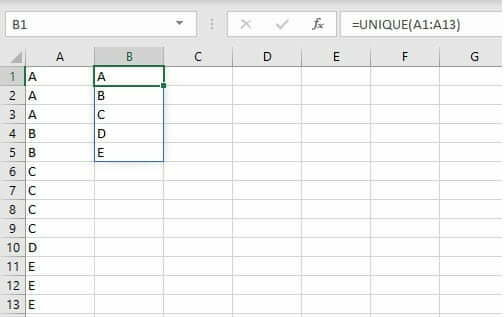I use Excel (O365) for data manipulation on a regular basis, as such I tend to have a need to use a wide range of formulas to process and manipulate that data. In this post, I wanted to discuss two (2) of the most useful formulas which I commonly use.

The first formula is unique, this returns a list of unique values from the range specified. The formula is:

=unique(range, by_column, Occurs_Only_Once)

In the above formula, only the range is mandatory. Typically the formula looks for unique values by row however, if you want to set it as looking for unique values by the column you set that to either 1 or true. The last variable that the function accepts is occurs_only_once, by default if you leave this out it will show all unique values but if you want to only show the values that are unique AND occur only once you can set this to 1 or TRUE.

In the below screenshot, I have shown an example of the unique function/formula. In this case, it searches through column A from row 1 through to row 13 and returns only the unique values in the form of an array.This is a useful function as you may have a list of values (i.e. categories, dates or so on) which you want to extract, so that you can start to use other formula's (i.e. sumifs, index/match), and rather than have to worry about duplicated values, the use of UNIQUE solves this problem.  In the above example, this creates a vertical list (by default) however there may be a case where you want to use the unique function, but instead of it running vertical you want it to run horizontal so that you can create your own table or something similar.

To change the orientation of the list, the most simple way is to use the transpose function. This works the same way as if you copy and paste special and use transpose, but it is within a function instead of copy and paste.  The transpose function is one of the easiest functions, it simply takes a range of cells or values (an array), and changes the direction.

=transpose(range or array)

If I wanted to transpose cells A1 to A4, I would use the function =transpose(A1:A4) however, because this is used in array functions you need to press CTRL + SHIFT + ENTER. In the earlier example, I am transposing a series of cells however, I can also use a formula within transpose, so I could do something like =transpose(unique(A1:A13)) then hit CTRL + SHIFT + ENTER and this would transpose from where I put that formula. This looks like the below:

Hopefully this little trick is useful, I use it on a regular basis to help me change the layout of many of my spreadsheets and hopefully it may be of use to you as well.